# circuitry-tools

Equivalent resistance and capacitance calculator for parallel/series circuits

View the Project on GitHub emanuelfeld/circuitry-tools

## Equivalent Circuit Calculator

Diagram the parallel/series* circuit of resistors or capacitors below as a comma-separated list of numbers and arrays. Numbers (integer or decimal) represent resistance or capacitance values. Using nested arrays, you can indicate parallel branches.

What are you calculating?

Resistance
Capacitance

Enter the circuit diagram

* For more complex topologies, you will need a different tool.

### Diagram Examples

Each example shows an image of a circuit segment (with resistors or capacitors), followed by the text ‘diagram’ you would use to represent it in the box above.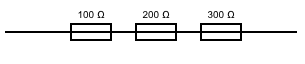``````100, 200, 300
``````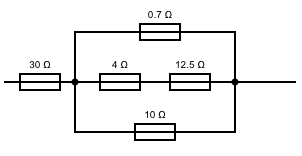``````30, [[0.7], [4, 12.5], ]
``````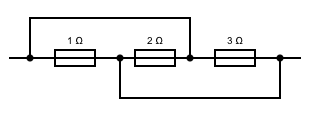``````[, , ]
``````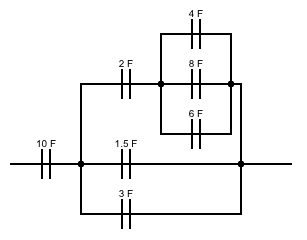``````10, [
[2, [
,
,

]
],
[1.5],

]
``````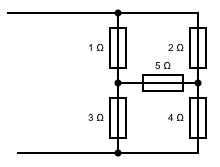No can do!